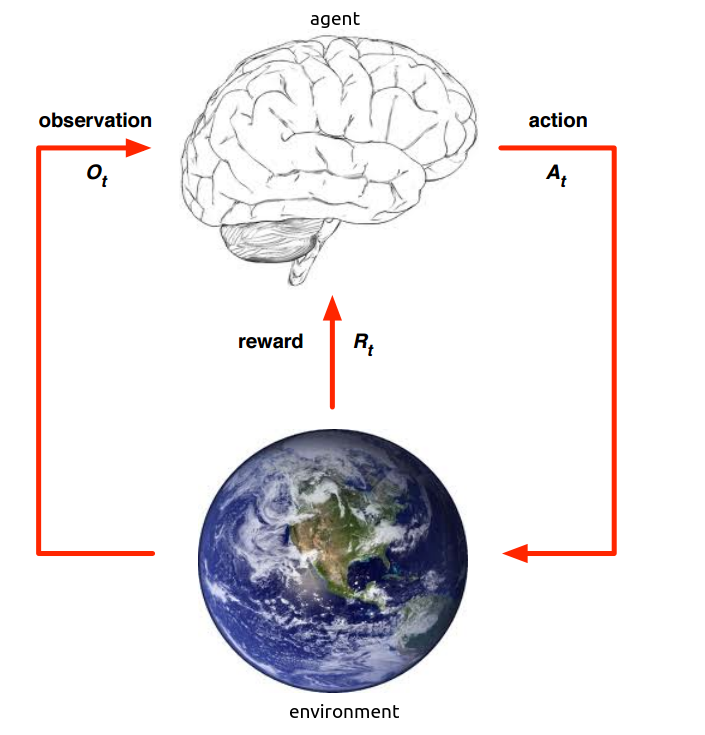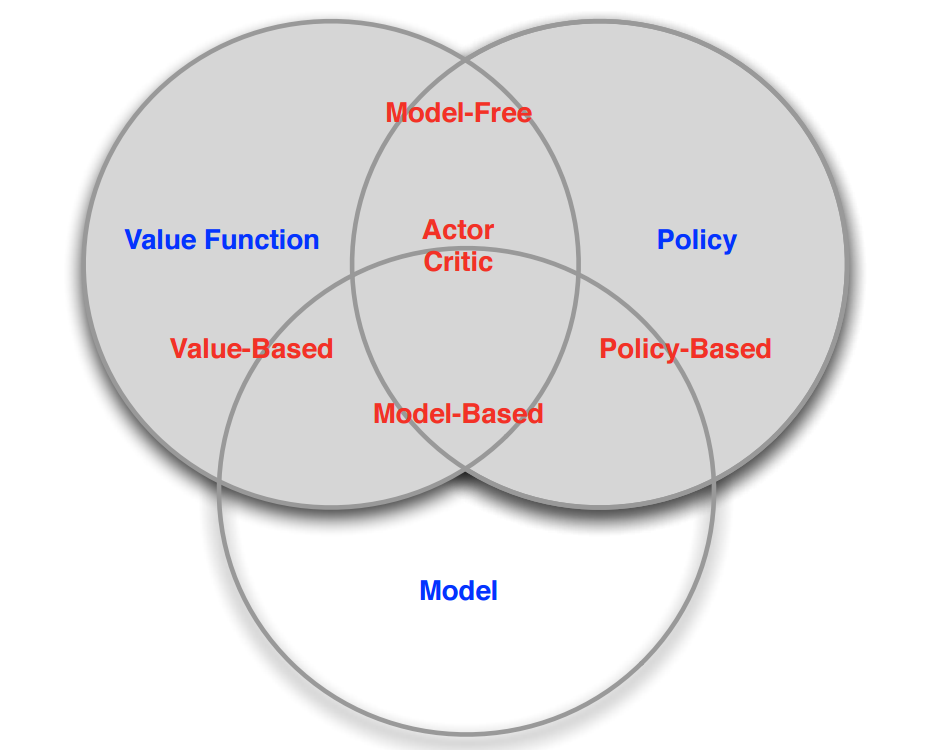【转载请注明出处】chenrudan.github.io2. 强化学习组成3. 一些变量

history是所有动作、状态、奖赏的序列，$H_t=A_1, O_1, R_1,…,A_t, O_t,R_t$

environment state，$S_t^e$，环境当前的状态，它反应了环境发生什么改变。这里需要明白的一点是环境自身的状态和环境反馈给agent的状态并不一定是相同的，例如机器人在走路时，当前的environment状态是一个确定的位置，但是它的camera只能拍到周围的景象，无法告诉agent具体的位置，而拍摄到的照片可以认为是对环境的一个observation，也就是说agent并不是总能知道环境是如何发生改变的，只能看到改变后的一个结果展示。

agent state，$S_t^a$，是agent的现在所处状态的表示，它可以是history的任何函数。

information(Markov) state，它包含了history的所有有用信息。一个状态$S_t$有马尔可夫性质是指下一个时刻的状态仅由当前状态决定，与过去状态无关。这里定义可以看出environment state是有马尔可夫性质的(这个概念不明白可以暂时不管)。

4. Agent的组成

Policy，它根据当前看到的observation来决定action，是从state到action的映射。有两种表达形式，一种是Deterministic policy即$a=\pi(s)$，在某种状态s下，一定会执行某个动作a。一种是Stochastic policy即$\pi(a|s)=p[A_t = a | S_t=s]$，它是在某种状态下执行某个动作的概率。

Value function，它预测了当前状态下未来可能获得的reward的期望。$V_{\pi}(s)=E_{\pi}[R_{t+1}+rR_{t+2}+…|S_t=s]$。用于衡量当前状态的好坏。

Model，预测environment下一步会做出什么样的改变，从而预测agent接收到的状态或者reward是什么。因而有两种类型的model，一种是预测下一个state的transition model即$P_{ss’}^a=p[S_{t+1}=s’|S_t = s,A_t=a]$，一种是预测下一次reward的reward model即$R_s^a=E[R_{t+1}|S_t=s, A_t=a]$